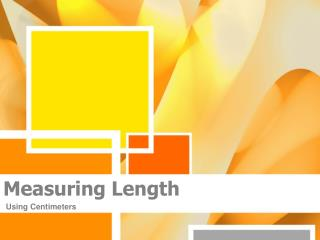DownloadDownload PresentationMeasuring Length

# Measuring Length

Télécharger la présentation## Measuring Length

- - - - - - - - - - - - - - - - - - - - - - - - - - - E N D - - - - - - - - - - - - - - - - - - - - - - - - - - -
##### Presentation Transcript

1. Measuring Length Using Centimeters

2. example • The line is 4.2 cm. • Start the ruler at zero. • The tiny spaces between the larger numbered lines are millimeters. • They are one tenth of a centimeter. • Notice the line is 4 whole centimeters and two tenths of centimeter.

3. Millimeters • Each centimeter has 10 smaller divisions. • These are millimeters. • 3.4 centimeters or 3.4 cm is the same as 3 centimeters and 4 millimeters.

4. Perimeter

5. Perimeter • Add the lengths of all the edges • This is the perimeter of a shape.